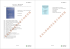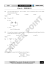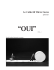# Variables and Patterns Review #2 - Ms

## Transcription

Variables and Patterns Review #2 - Ms
```Variables and Patterns Extra Practice –
1. Stefan said he had done an experiment similar to the jumping jack exercise. He had collected data on the number of deep knee bends
a person could do in 2 minutes. The graph below shows his data.
a. What are the two variables?
b. Make a table of Stefan’s data.
c. Describe in detail how the number of deep knee bends changes as time increases.
d. From Stefan’s data estimate the number knee bends he had done in 25 seconds and in 65 seconds. Explain how you made
those estimates.
2. The table shows some data Anita collected while walking.
Time (in minutes)
Distance(in miles)
0
0
5
0.5
10
1
15
1.25
a. What are the two variables?
b. Graph the data from the table on the axes below.
c. In what time periods did Anita make the most progress?
How does this show up in the table?
How does this show up in the graph?
d. In what time periods did Anita make the least progress?
How does this show up in the table?
How does this show up in the graph?
20
1.5
25
1.75
30
1.8
3. Teresa baby-sits for \$4.50 an hour.
a. Make a table showing how much money she will make over time.
b. Graph your data, label the axes and name the graph.
c. Does it make sense to connect the points on the graph? Why or why not?
e. If Teresa baby-sits 5.5 hours, how much will she earn? Explain your answer.
4. The three graphs below show the progress of a cyclist at different times during a ride.
For each graph, describe the rider’s progress over the time interval.
5. The graph below shows the number of cans of food collected by Mr. Darrow’s students on each of the five days of the school’s food
drive.
a. The graph shows the relationship between two variables. What are the variables?
b. On which day were the most cans of food collected?
How many cans were collected on that day?
c. What was the total number of cans collected over the 5 days? Explain your reasoning.
d. Does it make sense to connect the points with line segments? Explain your reasoning.
6. Dominic and Norm decide to save their money to go on a bike tour in their state. Dominic thinks he can save \$10 per week. Norm has
a. Make a table that will show the amount of money each boy will have over the next ten weeks if they stick to their plans.
b. Write a rule to show how much money each boy has for any number of weeks.
Dominic:
Norm:
c. Use the entries in your tables to graph each boy’s savings over time. Label the axes and name your graph. Use a different color to
graph the data for each boy.
d. Will the boys ever have the same amount of money at the same time? Explain your reasoning.
e. If the bike tour costs \$190, when will each boy have enough money to go on the tour?
7. The table shows some data Carmen collected during her swim team practice.
Number of breaths
Number of meters swum
0
0
1
5
2
8
3
12
4
15
5
17
a. What are the two variables?
b. Graph the data from the table on the axes below.
c. Does it make sense to connect the points? Explain your reasoning.
d. When did Carmen make the most progress?
i.
How does this show up in the table?
ii. How does this show up in the graph?
e. When did Carmen make the least progress?
i.
How does this show up in the table?
ii. How does this show up in the graph?
f.
How many breaths do you think she would take if she swam 50 meters?
6
20
7
24
8. The graph below shows data that Elizabeth collected while walking.
a. When does she make the most progress? Explain your reasoning.
b. When does she make the least progress? Explain your reasoning.
9. Each day the cafeteria workers at Edison Middle School start out with 400 cartons of milk. They collected some data and made the
following graph.
a.
What is the total number of cartons of milk sold?
b.
How many cartons were sold between noon and 12:30 p.m.?
c.
During what 15-minute time period(s) was the most milk sold?
d.
During what 15-minute time period(s) was the least amount of milk sold?
e.
Describe how the total number of cartons of milk available changed as the day progressed.
f.
Should the cafeteria workers have connected the points? Explain.
g.
Would a table be useful? Why or why not?
10. Mary and Juanita made the following graphs.
a. Did Mary and Juanita graph the same data set? Explain your reasoning.
b. Write a rule relating the number of ounces to the calories consumed from Mary’s graph.
11. Francisco was working on a problem on his graphing calculator. He saw the following two screens.
His partner made a sketch of the two screens. Then, he accidentally cleared the rule from the screen.
a. Write a rule that would re-create this graph and table.
b. Give a situation that could be represented by your rule.
12. The graph below shows the number of cans of food collected by Mr. Darrow’s students on each of the five days of the school’s holiday
food drive.
a. The graph shows the relationship between two variables. What are the variables?
b. On which day were the most cans of food collected? How many cans were collected on that day?
c. What total number of cans were collected over the 5 days? Explain your reasoning.
d. What is the mean number of cans collected over the five days? Explain your reasoning.
e. On this graph, does it make sense to connect the points with line segments? Explain your reasoning.
13. Andrew’s mother decided to keep a chart of the number of words his little sister Sarah could say at the end of each month from age 1
month to 24 months (2 years of age). Sarah did not say a word until 12 months, so from 1 to 11, Andrew’s mother just wrote 0.
a. Make a coordinate graph of these data. Which variable should be put on the x-axis and why? The y-axis?
b. Describe how the number of words Sarah can say changed as she got older (as the number of months passed).
c. During what month did Sarah learn to say the most words? The least (not counting from 1 to 11 months?
14. The Student Council of Metropolis Middle School recently voted on seven different proposals related to school activities. There are nine
students on the Student Council and each student must vote “yes” or “no” for each proposal. The number of “yes” votes for each
proposal were the following. Use the information in the table below to answer the following questions:
a. The above table shows a relationship between two variables. What are the variables?
b. Which variable is the independent variable and which is the dependent variable? Explain your reasoning.
c. Make a coordinate graph of the data in the table. Make sure to label your x-axis and y-axis with the correct independent or
dependent variable.
d. Now make a coordinate graph showing how many students voted “no” on each of the seven proposals. Explain how you find
the data for your graph and label the x-axis and y-axis with the appropriate independent or dependent variable.
15. Below is a chart of the water depth in a harbor during a typical 24-hour day. The water level rises and falls with the tide.
a. Make a coordinate graph of the data.
b. During which time interval(s) does the depth of the water increase the most?
c. During which time interval(s) does the depth of the water decrease the most?
d. Would it make sense to connect the points on the graph? Why or why not?
e. Is it easier to use the table or the graph to answer parts b and c? Explain.
16. A Newspaper included the graph below in a story about the amount of city land used for trash between 1990 and 1995.
a. The graph shows the relationship between two variables. What are they?
b. What is the difference between the least and greatest amount of land used for trash?
c. Between which two years did the area used for trash stay the same?
d. On this graph, what information is given by the lines connecting the points? Is this information necessarily accurate? Explain
e. In 2000, the total area available for trash was 120 square kilometers. Make a coordinate graph that shows the landfill area
remaining in each year from 2000 to 2005.
17. This table shows the fees charged for roller-blading at a roller rink.
a. Make a coordinate graph of these data.
b. Would it make sense to connect the points on your graph? Why or why not?
c. Using the table, describe the pattern of change in the total skating fee as the number of minutes increases. How is this pattern
shown in the graph?
18. A roller-blade supply store rents roller blades for \$2.50 per skater.
a. Using increments of 5 skaters, make a table showing the total rental charge for 0 to 50 skaters. Make a coordinate graph of
these data.
b. Compare the pattern of change in your table and graph with the patterns you found in the skating fees in question 3. Describe
any similarities and differences between the two sets of data.
19. The coordinate graph below shows the total monthly sales for the concession stand at the roller rink.
a. Use the graph to make a table of data showing the sales for each month.
b. The profit made by the concession stand is half of the sales. Make a table of data that shows the profit made by the
concession stand for each month.
c. Make a coordinate graph of the data from part b. Use the same scale used in the sales graph above. Describe how the sales
graph and the profit graph are similar and how they are different.
20. The three graphs below show the progress of a cyclist at different times during a ride. For each graph, describe the rider’s progress
over the time interval.
21. In (a)–(e), use symbols to express the rule as the equation. Use single letters to stand for the variables. Identify what each letter
represents.
a. The perimeter of a rectangle is twice its length plus twice its width.
b. The area of a triangle is one-half its base multiplied by its height.
c. Three big marshmallows are needed to make each s’more.
d. The number of quarters in an amount of money expressed in dollars is four times the number of dollars.
e. A half-cup of unpopped popcorn is needed to make 6 cups of popped popcorn.
22. The equation d = 44t represents the distance in miles covered, after traveling 44 miles per hour for t hours.
a. Make a table that shows the distance traveled, according to this equation, for every half hour between 0 and 4 hours.
b. Sketch a graph that shows the distance traveled between 0 and 4 hours.
c. If t = 2.5, what is d?
d. If d = 66, what is t?
e. Does it make sense to connect the points on this graph with line segments? Why or why not?
23. The number of students at Smithville Middle School is 21 multiplied by the number of teachers.
a. Use symbols to express the rule relating the number of students and the number of teachers as an equation. Use single letters
for your variables and explain what each letter represents.
b. If there are 50 teachers at Smithville Middle School, how many students attend the school?
c. If 1,260 students attend Smithville Middle School, how many teachers teach at the school?
24. The Student Council at Urbandale Middle School want to throw an end of the year party for students on the last day of school. They
decide to have breakfast catered and compare bids of two local catering companies. The Catering Crew charges \$8 per student for the
breakfast. Urbandale Catering Company charges a set fee of \$160 plus \$6 per student.
a. Make tables that show costs to have each company cater in cases where 20, 40, 60, 80, 100, and 120 students would attend.
b. Plot the (number of students, catering costs) on a graph. Use different colors or plotting symbols for points to show the two
catering companies.
c. Write equations relating total cost and number of students for each catering company.
If 60 students signed up to come to the breakfast, which company should the Student Council select? What if 100 students signed up
for the breakfast?
25. The tour operators thought it would be a good idea to get a souvenir T-shirt for each customer who went on the Ocean Bike Tour. They
found a company who would sell them shirts with their logo for \$6.95.
a. Make a table and a graph that show number of shirts and cost.
b. Would it make sense to connect the points on your graph with a line? Why or why not?
c. Write a rule using symbols to determine the T-shirt cost for any number of customers.
26. The number of hours of daylight in a day changes throughout the year. We say that the days are “shorter” in winter and “longer” in
summer. The following table shows the number of daylight hours in Chicago, Illinois, on a typical day during each month of the year
(January is month 1, and so on).
Describe the pattern relating the month and the number of daylight hours.
Month
1 (January)
2 (February)
3 (March)
4
5
6
7
8
9
10
11
12
Daylight hours
10.0
10.2
11.7
13.1
14.3
15.0
14.5
13.8
12.5
11.0
10.5
10.0
27. Dee bought a compact disc player with the money he earned working during his vacation. He checked some stores to find the cheapest
•
Taylor’s Department Store sells CDs for \$15.49 each.
•
Buyer’s Warehouse has an initial \$25 membership fee to shop there, then each CD would cost \$12.
a. Write a rule using symbols to determine the cost for any number of CDs at each store.
Taylor’s Department Store:
Multiple Choice
Identify the choice that best completes the statement or answers the question.
____
28.
a. 17
____ 29.
a. 20
____
b. 18
c. 16
d. 19
Approximately how many words had this student typed in 30 seconds?
b. 24
30.
a. 25.5
b.
c. 28
d. 34
If y = 12 + 3.5x, what is the value of y when x = 10?
b. 155
____ 31.
equation?
a.
____
What is the value of y when x is 68?
c. 47
d. 362
The equation c = 0.75t represents the total cost of tickets for carnival games. Which table best represents this
c.
d.
32.
Which problem situation matches the equation 6x = 90?
a. Hahn exercised 90 minutes each day for 6 days this month. What is the total number of hours, x,
that he exercised this month?
b. Tori charges \$6 per hour to cut lawns. What is the number of hours, x, that Tori worked if she
charged \$90 for her labor?
c. Lizzie drove a total of 90 miles this week. She drove 6 miles more than last week. What in the
number of miles, x, that Lizzie drove last week?
d. Gustav had saved \$90. He gave 6% to his six-year-old sister. What is the amount, x, that he has
left?
____
33.
a. 15
____
b. 53
34.
a. y = x +2
____
2
a.
b.
c.
d.
If y = x – 19, what is the value of x when y = 34?
35.
c. –15
Which equation shows the relationship between x and y shown in the graph?
b. y = 2x
c. y = 2x + 2
36.
d.
y= x
Which of the following best explains how the following pattern is formed?
3 5 8 13 …
Multiply each number by the number before it
Multiply by 2 and subtract 1
Add the previous two numbers together to get the new number
____
d. –53
Which graph best represents the table below?
a.
c.
b.
d.
Identify an equation that models the situation and find its solution.
____ 37.
A tomato plant was 9 inches tall when it was planted in June. When the first tomatoes were ripe, the plant was 42
inches tall. How many inches did the plant grow?
a.
c.
b.
d.
____
38.
Which graph most likely describes the distance a person walks in a 24-hour period?
a. Graph II
b. Graph III
c. Graph IV
d. Graph I
____ 39.
Which graph below could match the situation described?
A car traveling at 0 mi/h accelerates to 25 mi/h over the first 5 seconds. It maintains that speed for the next 5 seconds, and then
accelerates to 48 mi/h during the next 5 seconds.
c.
50
50
45
45
40
40
35
35
Speed (mi/h)
Speed (mi/h)
a.
30
25
20
30
25
20
15
15
10
10
5
5
0
2
4
6
8
10
12
0
14
2
4
Time (s)
6
8
10
12
14
12
14
Time (s)
d.
50
50
45
45
40
40
35
35
Speed (mi/h)
Speed (mi/h)
b.
30
25
20
30
25
20
15
15
10
10
5
5
0
2
4
6
8
10
Time (s)
12
14
0
2
4
6
8
10
Time (s)
____
40.
The graph shows the amount of gas in the tank of Sharon’s car during a trip to her mom’s house.
5:00
6:00
13
12
11
Gas (gallons)
10
9
8
7
6
5
4
3
2
1
0
7:00
8:00
Time (P.M.)
9:00
10:00
a. At one point Sharon started the trip. When did she do that?
b. Approximately how many gallons did she have when she started the trip?
a. 7:30 P.M; 6 gallons
c. 9:45 P.M; 15 gallons
b. 5:00 P.M.; 12 gallons
d. 7:00 P.M; 7 gallons
5
5
4.5
4.5
4
4
3.5
3.5
Distance (mi)
Distance (mi)
____ 41.
Select a graph for the situation. You wait for the express bus for 30 minutes, get on and ride the bus non-stop for 3
miles, and then walk another mile to your home.
a.
c.
3
2.5
2
3
2.5
2
1.5
1.5
1
1
0.5
0.5
0
0
0
6
12
18
24
30
36
42
48
54
60
0
6
12
18
Time (min)
24
30
36
42
48
54
60
42
48
54
60
Time (min)
d.
5
5
4.5
4.5
4
4
3.5
3.5
Distance (mi)
Distance (mi)
b.
3
2.5
2
3
2.5
2
1.5
1.5
1
1
0.5
0.5
0
0
0
6
12
18
24
30
36
Time (min)
42
48
54
60
0
6
12
18
24
30
36
Time (min)
Variables and Patterns Review #2
1.
ANS:
a. Number of seconds; total knee bends
b.
# of seconds
10
20
30
40
50
60
70
80
90
100
110
120
total knee bends
10
18
27
36
45
53
58
62
65
68
70
72
c. Stefan begins by doing approximately nine knee bends every ten seconds, but as time goes on the number of knee bends really
decreases down to about two knee bends for every ten seconds.
d. In 25 seconds, he should have done about 22 or 23 knee bends.
In 65 seconds, he should have done about 55 or 56 knee bends.
2.
ANS:
a. Time and Distance
b.
c. She made the most progress at the beginning walking 1 mile in the first 10 minutes. (A student may say 0 to 5 minutes or 5 to10
minutes.)
On the table, this shows up as the largest increase from one distance to the next. On the graph, this shows up as the steepest
line segment connecting the points.
d. Anita made the least progress from 25 minutes to 30 minutes walking only 0.05 of a mile. On the table, this shows up as the
smallest increase from one distance to the next. On the graph, this shows up as the least steep line segment connecting the points.
3.
ANS:
a.
b.
Time
1
2
3
4
5
6
7
8
Money
4.50
9.00
13.50
18
22.50
27
31.5
36
c.
Students may argue for or against connecting the dots. As they work longer hours then their money earned increases
indicating a continuous change but when people work part of an hour they are often paid for the full hour in which case it would not be
continuous.
d.
e.
\$24.75
4.
ANS:
a. As time passes, speed increases at a steady rate.
b. As time passes, speed decreases at a steady rate.
c. The person goes at a fast pace for a short time then continues on at a slower pace.
5.
ANS:
a. Days of collecting, Number of cans collected
b.
c. Day 1 78, Day 2 60, Day 3 60, Day 4 34, Day 5 63
d. No it does not make sense to connect the points (unless you just want to see the pattern.) You could not have collected partial
cans.
6.
ANS:
a.
Weeks
0
1
2
3
4
5
6
7
8
9
10
Dominic’s Money
0
10
20
30
40
50
60
70
80
90
100
Norm’s Money
25
32
39
46
53
60
67
74
81
88
95
b. From the directions students may write the rule in words or symbols. (Students will be more likely to give a rule in words since they
have not written symbolic rules with a constant while working this unit.)
Dominic -- Number of weeks times 10 equals total saved
10w = s
Norm -- Number of weeks times 7 plus 25 equals the total saved.
7w + 25 = s
c. Norm is graphed in blue with circles, and Dominic is in black with open rectangles.
d. No, not if they put their money in at the end of the week. At 8 weeks they will be
very close. Dominic will have \$80 and Norm will have \$81.
If they save parts of the amounts during the week, they could have the same
amount between the 8th and 9th weeks.
e. Dominic will have enough money in 19 weeks.
Will would have enough in 24 weeks
7.
ANS:
a. The two variables are the number of breaths and the number of meters swum.
b.
c. Answers will vary. Students may argue for or against connecting the points. Some may say that breathing is continuous, but in
swimming the breaths are more defined.
d. Carmen made the most progress during the first breath.
i. In the table the distance increases from 0 to 5 meters for the first breath. This is the largest between any two breaths.
ii. The distance between the origin and the corresponding point, (0, 5) is greatest on the graph.
e. The least progress is a change of 2 meters, which occurs between breaths 4 and 5.
i. In the table, this is the smallest distance between any two breaths.
ii. The corresponding points on the graph (4, 15) and (5, 17) are the closest two points on the graph.
f.
8.
ANS:
a.
Elizabeth makes the most progress at two different times-in the first 25 minutes and from 40 to 45 minutes. This progress is
shown on the graph by the steepest inclines.
b.
She makes the least progress from 25 to 40 minutes. This is shown by the flattest incline.
9.
ANS:
a.
b.
c.
Between 11:30 and 11:45 and between 12:45 and 1:00
d.
Between noon and 12:15, between 12:30 and 12:45, and between 1:15 and 1:30
e.
The number of milk cartons available during the day decreased rapidly with breaks at noon, 12:30, and 1:15.
f.
Possible answer: The points should not be connected because they cannot sell part of a milk carton.
g.
Answers will vary. Some students might say no because the graph gives a picture of what happened. Others might say yes
because it would be helpful to have more specific information especially since the y-axis has a fairly large scale.
10.
ANS:
a.
Yes they both graphed the same data. They used different scales. But the coordinates of the points are the same. For
example, (0, 0), (1, 80) … (2.5, 200) lie on both graphs.
b.
The equations for each graph are the same: y = 80x.
11.
ANS:
a. y = 4x
b. There are many possibilities.
y is the distance in miles for a walker who walks at an average rate of 4 mph.
y is the cost of buying tickets that cost \$4 each.
In a certain game a player receives 4 points for each win. y is the number of points a player wins after x wins.
12.
ANS:
a.
“Class period” is the independent variable and “number of cans” is the dependent variable; the number of cans depends on
the class period.
b. 1st period collected the most cans of food, about 75.
c. 75 + 60 + 60 + 35 + 70 = 300 cans of food.
d.
The information provided by the graph shows that 5 classes collected a total of 300 cans of food, so the average number of
cans collected by each class would be
= 60.
e.
The graph does not indicate the number of students in each of Mr. Darrow’s classes. The fact that the average number of
cans donated per student is higher in second period than fifth period, with fifth period donating more total cans means that there must
be more students in Mr. Darrows fifth-period class than in his second-period class.
13.
ANS:
a.
b. Answers will vary. It is important to give students some examples of complete and thoughtful responses early in this unit so they
have a sense of what is expected of them. Possible response: Sarah learned no words between the first month and the eleventh. She
learned her first word during the 12th month and learned only a few (about 1 per month) until the 16th month. At the end of 16 months,
Sarah knows 7 words and continues to learn new words at more than 1 per month from then on. Between 16 months and 19th months,
Sarah learns an increasing number of new words each month (3, 5, 6) and then the number of new words she learns each months
decreases in the 20th and 21st month (she learns 4 and then 2 new words). The number of new words Sarah learns increases rapidly
in the 22nd month (17 new words). This might represent a developmental milestone. She then learns less in the 23th (8) and slightly
more in the 24th month (11).
c. She learned the most in the 22nd month (17 new words). She learned the least, not counting between 1 and 11 months, between
the 12th and 13th month when she did not learn any new words. She still only knew 1 word in the 13th month.
14.
ANS:
a.
The variables are “proposal” and “number of yes votes.”
b. “Proposal” is the independent variable and “yes votes” is the dependent variable; the number of “yes votes” is determined by which
“proposal” is being voted on.
c. The graph should look like this:
d. Since 9 Student Council members voted on each proposal and each member must vote yes or no (i.e., no abstentions), the
number of “no” votes for each proposal would be 9 – (# of yes votes). A coordinate graph of the data would look like this:
15.
ANS:
a.
b.
From 14 hours after midnight to 15 hours after midnight, the depth increases by
the greatest amount, 1.4 meters.
c.
Between 9 and 10 hours after midnight and between 22 and 23 hours after
midnight, the depth decreases by the greatest amount, 1.2 meters.
d. It makes sense to connect the points because the depth is changing continuously.
e. Possible answer: It is easier to use the table because you can read the exact values.
16.
ANS:
a. Year and land area in square kilometers
c. Between 1990 and 1991 and between 1993 and 1994
d. Possible answer: The information shows a constant change in the area
used for landfill from one year to the next. It isn’t necessarily accurate because
we do not know the details of how the landfill “grew” from one year to the next.
e.
17.
ANS:
a.
b. Possible answer: It would make sense to connect the points on the graph if
there are partial fees for minutes between half hours.
c.
The cost increases by \$3.50 for each additional half hour of skating.
On the graph, this is shown by a straight-line pattern going up as we read from
left to right. The values on the “Cost” axis increase by \$3.50 for each increase
of 30 on the “Minutes” axis.
18.
ANS:
a.
b.
Possible answer: Both tables show a constant increase in the y-values as the x-values increase by a fixed amount. The points
on both graphs follow a straight-line pattern.
19.
ANS:
a, b.
c. The graph of the profit is similar to the sales graph except that each y-coordinate in the profit graph is exactly half the value of the
y-coordinate in the total sales graph.
20.
ANS:
a.
The graph shows the cyclist’s speed constantly increasing.
b. The graph shows the cyclist’s speed constantly decreasing.
c. The graph shows the cyclist’s speed increasing and then leveling off.
21.
ANS:
a.
p is perimeter, l is length, w is width: p = 2l + 2w
b.
A is area, b is base, h is height: A =
bh (
b
h)
c. s is number of s'mores, m is number of marshmallows: s = 3m (3 m)
d. q is the number of quarters, D is the amount of money in dollars: q = 4D (4 D)
e. u is cups of unpopped popcorn, p is cups of popped popcorn: p = 12u (12 u)
22.
ANS:
a.
b.
c.
d.
e.
d = 110
t = 1.5
It makes sense to connect points because the distance increases at a constant rate.
23.
a.
b.
c.
ANS:
S is number of students, T is number of teachers: S = 21 T (21T)
S = 21 50 = 1050 students
If 1,260 = 21 T, what number must 21 be multiplied by to get a product of 1,260 T = 60 teachers.
24.
ANS:
a.
b.
c. For Catering Crew: C = 8n (where n is number of students and C is
total cost).
For Urbandale Catering Company: C = 150 + 6n (where n is number of
students and C is total cost).
25.
ANS:
b. Answers will vary. The points should not be connected because you cannot
purchase a portion of a shirt. However, some students may make the point that the cost
grows steadily so you could connect the points to see the pattern.
c.
26.
6.95s = c, where s is the number of shirts and c is the cost of the shirts
ANS:
In January, the number of daylight hours is only about 10. Through the months from January to June the number of daylight hours
increases. Then in July the number of daylight hours starts decreasing and by December is back to about 10 hours of daylight per day.
The greatest increase between months is in the early spring, February–March. And the faster decrease is in in the early fall, around
September to October.
27.
ANS:
a. Some students will start with a table to make sure the rules are correct.
Taylor’s Department Store
c = \$15.49n
c = \$12n + \$25
MULTIPLE CHOICE
28.
29.
30.
31.
32.
33.
34.
35.
36.
37.
38.
39.
40.
41.
ANS:
ANS:
ANS:
ANS:
ANS:
ANS:
ANS:
ANS:
ANS:
ANS:
ANS:
ANS:
ANS:
ANS:
A
C
C
A
B
B
B
D
D
A
C
A
B
D
|
```

### November 30, 2006 Update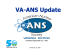### SOLUTION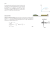### 3. JEE-main Physics 2015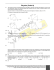### Application Brochure_16.cdr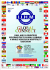### Solid Waste Flyer.indd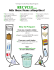### Cheddar Perron extra-fort 2 ans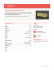### Presentation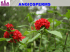### Cheddar Perron Le Doyen 4 ans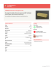### a-one xavier`s school - A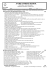### Find the structure for the following molecules: Problem 1.### Ohio State B - Collegiate Quizbowl Packet Archive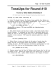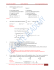### Mobb Deep, QB`s Finest - Collegiate Quizbowl Packet Archive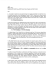### California Association of Neurological Surgeons Annual Meeting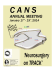### Message from Cindy L. December Birthdays WSL 2011 Door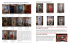### Untitled - Student Resources Centre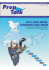### Student Notes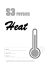### Untitled - Student Resources Centre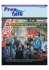### fasting - Islamic Laws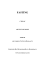### Robert Eisberg-Quantum Physics-Problem